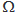# Statement-1: The temperature dependence of resistance is usually given as R = R0(1 + α∆t). The resistance of a wire changes from 100  ...

Statement-1: The temperature dependence of resistance is usually given as R = R0(1 + α∆t). The resistance of a wire changes from 100to 150when its temperature is increased from 27°C to 227°C. This implies that α = 2.5 × 10-3/°C

Statement-2: R = R0(1 + α∆t) is valid only when the change in the temperature ∆T is small and ∆R = (R – R0) << R0.

Choose one that best describes the two statements.

Anonymous User Physics Current Electricity 07 May, 2020 60 views